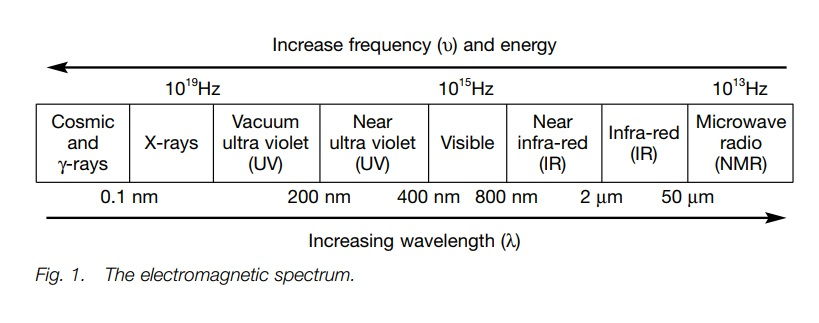Home | | Organic Chemistry | Spectroscopy

# SpectroscopyThe absorption or emission of energy from electromagnetic radiation is involved in IR, vis/uv and nmr spectroscopy. Mass spectroscopy involves measuring the deflection of ions in a magnetic field.

SPECTROSCOPY

## Introduction

The absorption or emission of energy from electromagnetic radiation is involved in IR, vis/uv and nmr spectroscopy. Mass spectroscopy involves measuring the deflection of ions in a magnetic field.

Electromagnetic radiation has the properties of both a wave and a particle. The former can be defined by wavelength and frequency, which in turn define the energy. Energy is proportional to frequency and inversely pro-portional to wavelength.

## Introduction

There are three important spectroscopic methods used in the analysis of organic compounds which involve the use of electromagnetic radiation. These are visible/ultra violet (vis/uv), infra-red (IR) and nuclear magnetic resonance (nmr) spectroscopy. These methods measure the absorption or emission of energy from electromagnetic radiation arising from different regions of the electromagnetic spectrum (Fig. 1). In contrast, mass spectroscopy measures the deflection of ions in a magnetic field.Electromagnetic radiation has the properties of both a wave and a particle. The latter can be described in terms of quanta or photons. The former can be described by wavelength (λ) – the distance between the crests of different waves, and frequency (υ) – the number of waves that pass a given point each second.Frequency is measured in hertz (Hz), which is the same as cycles per second. The energy of electromagnetic radiation is related to frequency and wavelength by the following equation:where h = Planck’s constant (6.63 × 1034 J s1), and c is the velocity of light (2.99792458 × 108 m s1).

Therefore, the higher the frequency of radiation, the higher the energy.Con-versely, the higher the wavelength, the lower the energy. Thus in the visible spec-trum, violet light (λ = 400 nm) has a higher energy than red light (λ = 750 nm).

Study Material, Lecturing Notes, Assignment, Reference, Wiki description explanation, brief detail

Related Topics